# 【BP预测】基于Logistic混沌映射改进的原子搜索算法优化BP神经网络实现数据预测matlab源码

## 一、 BP神经网络预测算法简介

### 1.1 受相关指标影响的BP神经网络算法原理

1）输入层：相当于人的五官，五官获取外部信息，对应神经网络模型input端口接收输入数据的过程。
2）隐含层：对应人的大脑，大脑对五官传递来的数据进行分析和思考，神经网络的隐含层hidden Layer对输入层传来的数据x进行映射，简单理解为一个公式hiddenLayer_output=F(w*x+b)。其中，w、b叫做权重、阈值参数，F()为映射规则，也叫激活函数，hiddenLayer_output是隐含层对于传来的数据映射的输出值。换句话说，隐含层对于输入的影响因素数据x进行了映射，产生了映射值。
3）输出层：可以对应为人的四肢，大脑对五官传来的信息经过思考（隐含层映射）之后，再控制四肢执行动作（向外部作出响应）。类似地，BP神经网络的输出层对hiddenLayer_output再次进行映射，outputLayer_output=w *hiddenLayer_output+b。其中，w、b为权重、阈值参数，outputLayer_output是神经网络输出层的输出值（也叫仿真值、预测值）（理解为，人脑对外的执行动作，比如婴儿拍打桌子）。
4）梯度下降算法：通过计算outputLayer_output和神经网络模型传入的y值之间的偏差，使用算法来相应调整权重和阈值等参数。这个过程，可以理解为婴儿拍打桌子，打偏了，根据偏离的距离远近，来调整身体使得再次挥动的胳膊不断靠近桌子，最终打中。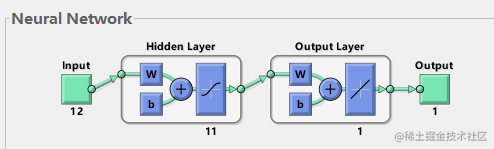## 二、原子搜索算法

1.原子优化算法原理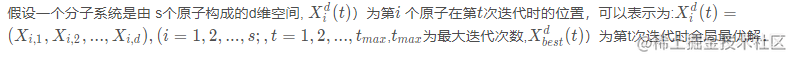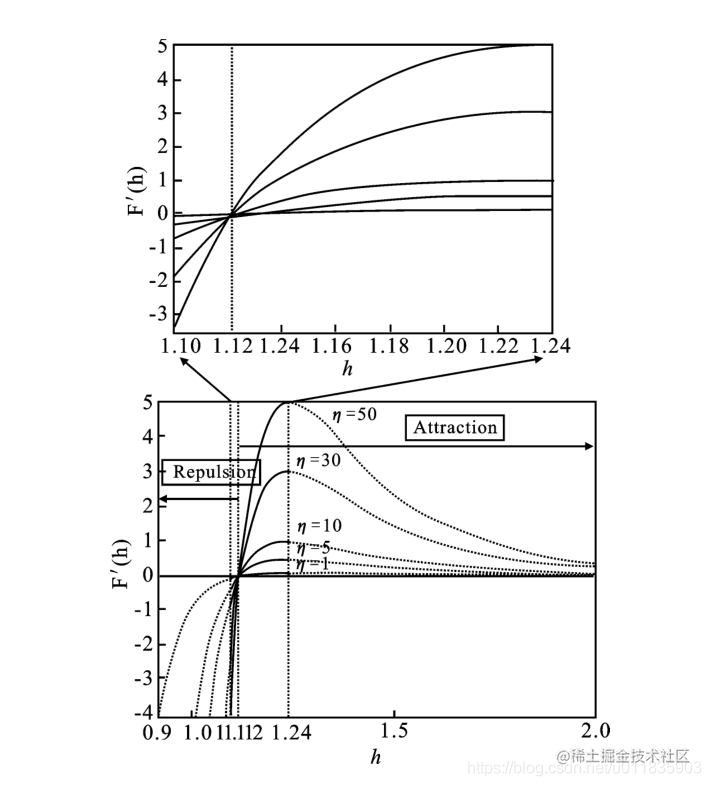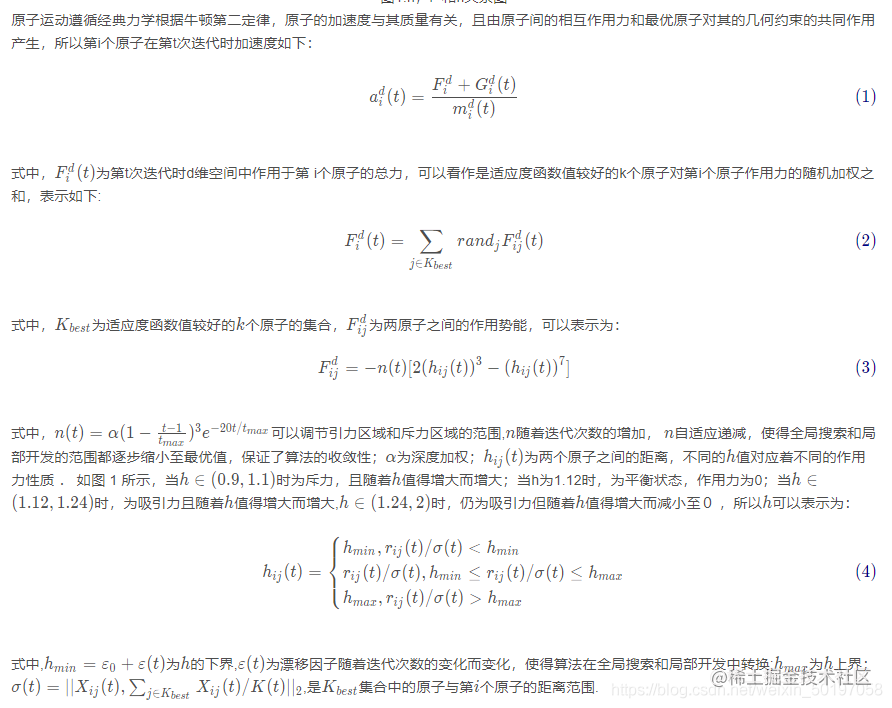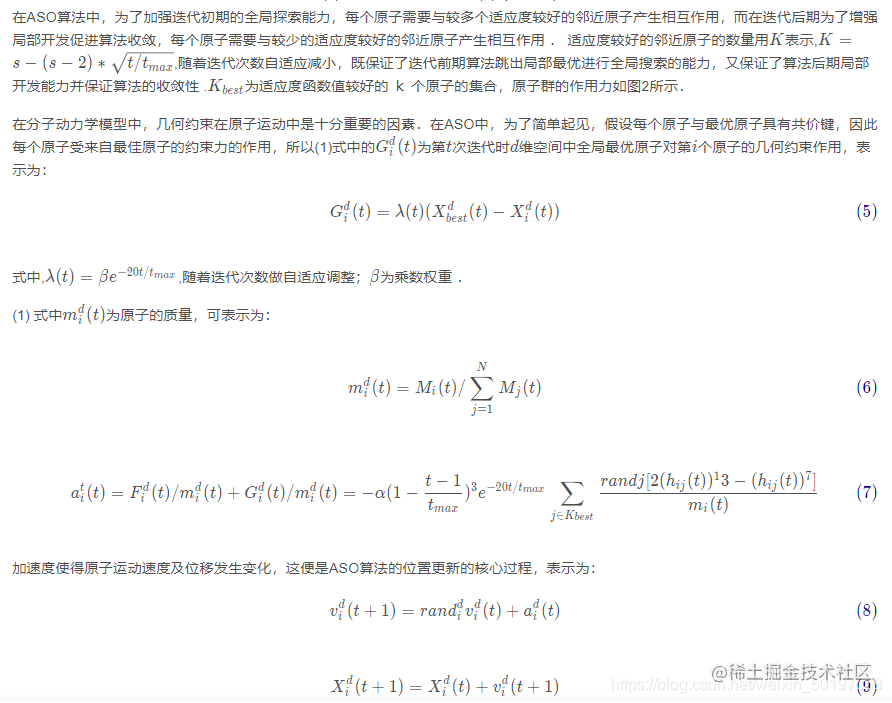## 三、ASO优化BP神经网络的步骤

Step1：初始化BP神经网络的权值和阈值
Step2：计Logistic混沌映射改进的原子搜索优化算法的决策变量长度，选取均方误差作为优化的目标函数。
Step3：设置算法停止准则，使用遗传优化算法优化神经网络的权值和阈值参数。
Step4：将优化得到的权值和阈值参数赋给BP神经网络。
Step5：优化后的BP神经网络训练与测试，与优化前的BP神经网络进行误差分析和精度对比。

## 三、演示代码

``````%--------------------------------------------------------------------------
% Atom Search Optimization.
function [X_Best,Fit_XBest,Functon_Best]=ASO(alpha,beta,Fun_Index,Atom_Num,Max_Iteration)

% Dim: Dimension of search space.
% Atom_Pop: Population (position) of atoms.
% Atom_V:  Velocity of atoms.
% Acc: Acceleration of atoms.
% M: Mass of atoms.
% Atom_Num: Number of atom population.
% Fitness: Fitness of atoms.
% Max_Iteration: Maximum of iterations.
% X_Best: Best solution (position) found so far.
% Fit_XBest: Best result corresponding to X_Best.
% Functon_Best: The fitness over iterations.
% Low: The low bound of search space.
% Up: The up bound of search space.
% alpha: Depth weight.
% beta: Multiplier weight

alpha=50;
beta=0.2;

Iteration=1;
[Low,Up,Dim]=Test_Functions_Range(Fun_Index);

% Randomly initialize positions and velocities of atoms.
if size(Up,2)==1
Atom_Pop=rand(Atom_Num,Dim).*(Up-Low)+Low;
Atom_V=rand(Atom_Num,Dim).*(Up-Low)+Low;
end

if size(Up,2)>1
for i=1:Dim
Atom_Pop(:,i)=rand(Atom_Num,1).*(Up(i)-Low(i))+Low(i);
Atom_V(:,i)=rand(Atom_Num,1).*(Up(i)-Low(i))+Low(i);
end
end

% Compute function fitness of atoms.
for i=1:Atom_Num
Fitness(i)=Test_Functions(Atom_Pop(i,:),Fun_Index,Dim);
end
Functon_Best=zeros(Max_Iteration,1);
[Max_Fitness,Index]=min(Fitness);
Functon_Best(1)=Fitness(Index);
X_Best=Atom_Pop(Index,:);

% Calculate acceleration.
Atom_Acc=Acceleration(Atom_Pop,Fitness,Iteration,Max_Iteration,Dim,Atom_Num,X_Best,alpha,beta);

% Iteration
for Iteration=2:Max_Iteration
Functon_Best(Iteration)=Functon_Best(Iteration-1);
Atom_V=rand(Atom_Num,Dim).*Atom_V+Atom_Acc;
Atom_Pop=Atom_Pop+Atom_V;

for i=1:Atom_Num
% Relocate atom out of range.
TU= Atom_Pop(i,:)>Up;
TL= Atom_Pop(i,:)<Low;
Atom_Pop(i,:)=(Atom_Pop(i,:).*(~(TU+TL)))+((rand(1,Dim).*(Up-Low)+Low).*(TU+TL));
%evaluate atom.
Fitness(i)=Test_Functions(Atom_Pop(i,:),Fun_Index,Dim);
end
[Max_Fitness,Index]=min(Fitness);

if Max_Fitness<Functon_Best(Iteration)
Functon_Best(Iteration)=Max_Fitness;
X_Best=Atom_Pop(Index,:);
else
r=fix(rand*Atom_Num)+1;
Atom_Pop(r,:)=X_Best;
end

% Calculate acceleration.
Atom_Acc=Acceleration(Atom_Pop,Fitness,Iteration,Max_Iteration,Dim,Atom_Num,X_Best,alpha,beta);
end

Fit_XBest=Functon_Best(Iteration);## 四、仿真结果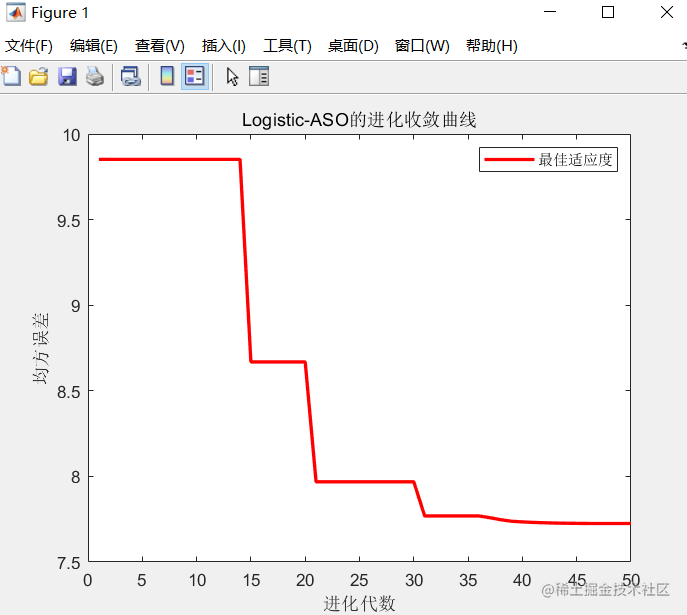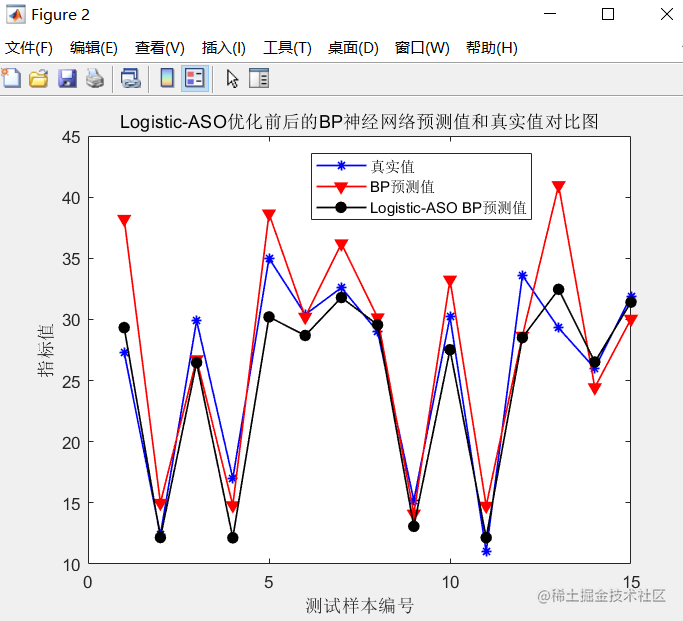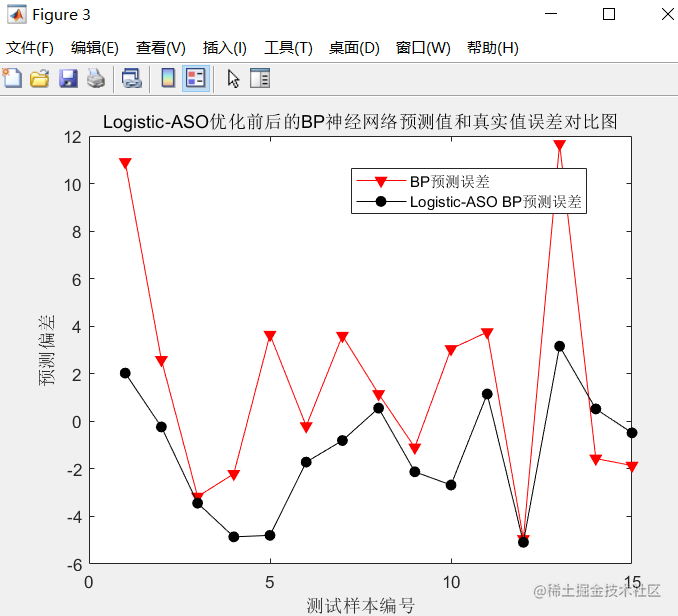## 五、参考文献及代码私信博主

《基于BP神经网络的宁夏水资源需求量预测》